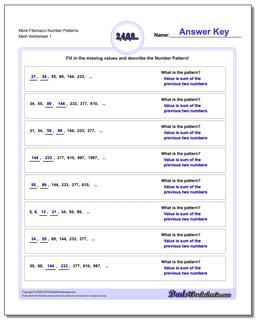# Math Worksheets: Number Patterns: Number Patterns: More Fibonacci Number Patterns## More Fibonacci Number Patterns

PropertyValue
DescriptionMore Fibonacci Number Patterns: Fibonacci and Fibonacci-like number pattern problems, where the next values in a sequence are dependent on prior values.
Resource TypeWorksheet